# 2 Types & Operations Written by Matt Galloway

Now that you know how to perform basic operations and manipulate data using these operations, it’s time to learn more about types. Formally, a type describes a set of values and the operations that can be performed on them. In this chapter, you’ll learn about handling different types, including strings that allow you to represent text. You’ll learn about converting between types and you’ll also be introduced to type inference which makes your life as a programmer a lot simpler. Finally, you’ll learn about tuple types, which allow you to group values of any type together.

## Type conversion

Sometimes you’ll have data in one format and need to convert it to another. The naïve way to attempt this would be like so:

``````var integer: Int = 100
var decimal: Double = 12.5
integer = decimal
``````

Swift will complain if you try to do this and spit out an error on the third line:

`Cannot assign value of type 'Double' to type 'Int'`

Some programming languages aren’t as strict and will perform conversions like this silently. Experience shows this kind of silent, automatic conversion is a source of software bugs and often hurts performance. Swift disallows you from assigning a value of one type to another and avoids these issues.

Remember, computers rely on us programmers to tell them what to do. In Swift, that includes being explicit about type conversions. If you want the conversion to happen, you have to say so!

Instead of simply assigning, you need to say that you want to convert the type explicitly. You do it like so:

``````integer = Int(decimal)
``````

The assignment on the third line now tells Swift unequivocally that you want to convert from the original type, `Double`, to the new type, `Int`.

Note: In this case, assigning the decimal value to the integer results in a loss of precision: The `integer` variable ends up with the value `12` instead of `12.5`. This is why it’s important to be explicit. Swift wants to make sure you know what you’re doing and that you may end up losing data by performing the type conversion.

### Operators with mixed types

So far, you’ve only seen operators acting independently on integers or doubles. But what if you have an integer that you want to multiply by a double?

You might think you could do it like this:

``````let hourlyRate: Double = 19.5
let hoursWorked: Int = 10
let totalCost: Double = hourlyRate * hoursWorked
``````

If you try that, you’ll get an error on the final line:

`Binary operator '*' cannot be applied to operands of type 'Double' and 'Int'`

This is because in Swift, you can’t apply the `*` operator to mixed types. This rule also applies to the other arithmetic operators. It may seem surprising at first, but Swift is being rather helpful.

Swift forces you to be explicit about what you mean when you want an `Int` multiplied by a `Double`, because the result can be only one type. Do you want the result to be an `Int`, converting the `Double` to an `Int` before performing the multiplication? Or do you want the result to be a `Double`, converting the `Int` to a `Double` before performing the multiplication?

In this example, you want the result to be a `Double`. You don’t want an `Int`, because in that case, Swift would convert the `hourlyRate` constant into an `Int` to perform the multiplication, rounding it down to 19 and losing the precision of the `Double`.

You need to tell Swift you want it to consider the `hoursWorked` constant to be a `Double`, like so:

``````let totalCost: Double = hourlyRate * Double(hoursWorked)
``````

Now, each of the operands will be a `Double` when Swift multiplies them, so `totalCost` is a `Double` as well.

### Type inference

Every variable or constant you’ve seen in this book so far includes a type annotation. You may be asking yourself why you need to bother writing the `: Int` and `: Double`, since the right-hand side of the assignment is already an `Int` or a `Double`. It’s redundant, to be sure; your crazy-clever brain can see this without too much work.

It turns out the Swift compiler can deduce this as well. It doesn’t need you to tell it the type all the time — it can figure it out on its own. This is done through a process called type inference. Not all programming languages have this, but Swift does, and it’s a key component of Swift’s power as a language.

So, you can simply drop the type in most places where you see one.

For example, consider the following constant declaration:

``````let typeInferredInt = 42
``````

Sometimes it’s useful to check the inferred type of a variable or constant. You can do this in a playground by holding down the Option key and clicking on the variable or constant’s name. Xcode will display a popover like this: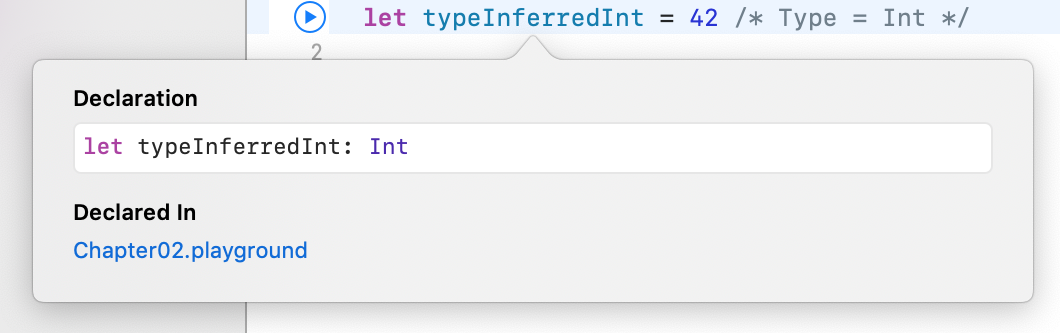Xcode tells you the inferred type by giving you the declaration you would have had to use if there were no type inference. In this case, the type is `Int`.

It works for other types, too:

``````let typeInferredDouble = 3.14159
``````

Option-clicking on this reveals the following: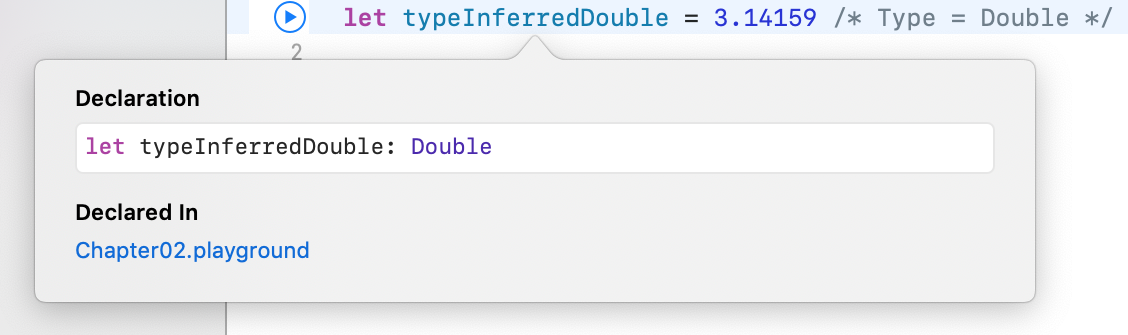You can see from this that type inference isn’t magic. Swift is simply doing what your brain does very easily. Programming languages that don’t use type inference can often feel verbose, because you need to specify the often obvious type each time you declare a variable or constant.

Note: In later chapters, you’ll learn about more complex types where sometimes Swift can’t infer the type. That’s a pretty rare case though, and you’ll see type inference used for most of the code examples in this book — except in cases where we want to highlight the type for you.

Sometimes you want to define a constant or variable and ensure it’s a certain type, even though what you’re assigning to it is a different type. You saw earlier how you can convert from one type to another. For example, consider the following:

``````let wantADouble = 3
``````

Here, Swift infers the type of `wantADouble` as `Int`. But what if you wanted `Double` instead?

The first thing you could do is the following:

``````let actuallyDouble = Double(3)
``````

This is like you saw before with type conversion.

Another option would be to not use type inference at all and do the following:

``````let actuallyDouble: Double = 3
``````

There is a third option, like so:

``````let actuallyDouble = 3 as Double
``````

This uses a new keyword you haven’t seen before, `as`. It also performs a type conversion, and you’ll see it again later in this book.

Note: Literal values like `3` don’t have a type. It’s only when using them in an expression or assigning them to a constant or variable that Swift infers a type for them.

A literal number value that doesn’t contain a decimal point can be used as an `Int` as well as a `Double`. This is why you’re allowed to assign the value `3` to constant `actuallyDouble`.

Literal number values that do contain a decimal point cannot be integers. This means we could have avoided this entire discussion had we started with:

`let wantADouble = 3.0`

Sorry! :]

### Mini-exercises

1. Create a constant called `age1` and set it equal to `42`. Create a constant called `age2` and set it equal to `21`. Check using Option-click that the type for both has been inferred correctly as `Int`.
2. Create a constant called `avg1` and set it equal to the average of `age1` and `age2` using the naïve operation `(age1 + age2) / 2`. Use Option-click to check the type and check the result of `avg1`. Why is it wrong?
3. Correct the mistake in the above exercise by converting `age1` and `age2` to type `Double` in the formula. Use Option-click to check the type and check the result of `avg1`. Why is it now correct?

## Strings

Numbers are essential in programming, but they aren’t the only type of data you need to work with in your apps. Text is also an extremely common data type used to represent things like people’s names, their addresses, or even the words of a book. All of these are examples of text that an app might need to handle.

Most computer programming languages store text in a data type called a string. This chapter introduces you to strings, first by giving you background on the concept of strings and then by showing you how to use them in Swift.

### How computers represent strings

Computers think of strings as a collection of individual characters. In Chapter 1 of this book, you learned that numbers are the language of CPUs, and all code, in whatever programming language, can be reduced to raw numbers. Strings are no different!

That may sound very strange. How can characters be numbers? At its base, a computer needs to translate a character into the computer’s own language, and it does so by assigning each character a different number. This forms a two-way mapping from character to number that is called a character set.

When you press a character key on your keyboard, you are actually communicating the number of the character to the computer. Your word processor application converts that number into a picture of the character and finally, presents that picture to you.

### Unicode

In isolation, a computer is free to choose whatever character set mapping it likes. If the computer wants the letter a to equal the number 10, then so be it. But when computers start talking to each other, they need to use a common character set.

If two computers used different character sets, then when one computer transferred a string to the other, they would end up thinking the strings contained different characters.

There have been several standards over the years, but the most modern standard is Unicode. It defines the character set mapping that almost all computers use today.

Note: You can read more about Unicode at its official website, http://unicode.org/.

As an example, consider the word cafe. The Unicode standard tells us that the letters of this word should be mapped to numbers like so:The number associated with each character is called a code point. So in the example above, c uses code point 99, a uses code point 97, and so on.

Of course, Unicode is not just for the simple Latin characters used in English, such as c, a, f and e. It also lets you map characters from languages around the world. The word cafe, as you’re probably aware, is derived from French, in which it’s written as café. Unicode maps these characters like so:And here’s an example using Chinese characters (this, according to Google translate, means “Computer Programming”):You’ve probably heard of emojis, which are small pictures you can use in your text. These pictures are, in fact, just normal characters and are also mapped by Unicode. For example:This is only two characters. The code points for these are very large numbers, but each is still only a single code point. The computer considers these as no different than any other two characters.

Note: The word “emoji” comes from Japanese, where “e” means picture and “moji” means character.

## Strings in Swift

Swift, like any good programming language, can work directly with characters and strings. It does so through the data types `Character` and `String`, respectively. In this section, you’ll learn about these data types and how to work with them.

### Characters and strings

The `Character` data type can store a single character. For example:

``````let characterA: Character = "a"
``````

This stores the character a. It can hold any character — even an emoji:

``````let characterDog: Character = "🐶"
``````

But this data type is designed to hold only single characters. The `String` data type, on the other hand, stores multiple characters. For example:

``````let stringDog: String = "Dog"
``````

It’s as simple as that! The right-hand side of this expression is what’s known as a string literal; it’s the Swift syntax for representing a string.

Of course, type inference applies here as well. If you remove the type in the above declaration, then Swift does the right thing and makes the `stringDog` a `String` constant:

``````let stringDog = "Dog" // Inferred to be of type String
``````

Note: There’s no such thing as a character literal in Swift. A character is simply a string of length one. However, Swift infers the type of any string literal to be `String`, so if you want a `Character` instead, you must make the type explicit.

### Concatenation

You can do much more than create simple strings. Sometimes you need to manipulate a string, and one common way to do so is to combine it with another string.

In Swift, you do this in a rather simple way: by using the addition operator. Just as you can add numbers, you can add strings:

``````var message = "Hello" + " my name is "
let name = "Matt"
message += name // "Hello my name is Matt"
``````

You need to declare `message` as a variable rather than a constant because you want to modify it. You can add string literals together, as in the first line, and add string variables or constants together, as in the last line.

It’s also possible to add characters to a string. However, Swift’s strictness with types means you have to be explicit when doing so, just as you have to be when you work with numbers if one is an `Int` and the other is a `Double`.

To add a character to a string, you do this:

``````let exclamationMark: Character = "!"
message += String(exclamationMark) // "Hello my name is Matt!"
``````

With this code, you explicitly convert the `Character` to a `String` before you add it to `message`.

### Interpolation

You can also build up a string by using interpolation, which is a special Swift syntax that lets you build a string in a way that’s easy to read:

``````message = "Hello my name is \(name)!" // "Hello my name is Matt!"
``````

As I’m sure you’ll agree, this is much more readable than the previous section’s example. It’s an extension of the string literal syntax, whereby you replace certain parts of the string with other values. You enclose the value you want to insert in parentheses preceded by a backslash.

This syntax works in the same way to build a string from other data types, such as numbers:

``````let oneThird = 1.0 / 3.0
let oneThirdLongString = "One third is \(oneThird) as a decimal."
``````

Here, you use a `Double` in the interpolation. At the end of this code, your `oneThirdLongString` constant will contain the following:

One third is 0.3333333333333333 as a decimal.

Of course, it would take infinite characters to represent one third as a decimal, because it’s a repeating decimal. String interpolation with a `Double` gives you no way to control the precision of the resulting string. This is an unfortunate consequence of using string interpolation: It’s simple to use, but offers no ability to customize the output.

### Multi-line strings

Swift has a neat way to express strings that contain multiple lines. This can be rather useful when you need to put a very long string in your code.

You do it like so:

``````let bigString = """
You can have a string
that contains multiple
lines
by
doing this.
"""
print(bigString)
``````

The three double-quotes signify that this is a multi-line string. Handily, the first and final new lines do not become part of the string. This makes it more flexible as you don’t have to have the three double-quotes on the same line as the string.

In the case above, it will print the following:

``````You can have a string
that contains multiple
lines
by
doing this.
``````

Notice that the two-space margin in the multiline string literal is stripped out of the result. Swift looks at number of leading spaces on the final three double-quotes line. Using this as a baseline, Swift requires that all lines above it have at least that much space so it can remove it from each line. This lets you format your code with pretty indentation without effecting the output.

### Mini-exercises

1. Create a string constant called `firstName` and initialize it to your first name. Also create a string constant called `lastName` and initialize it to your last name.
2. Create a string constant called `fullName` by adding the `firstName` and `lastName` constants together, separated by a space.
3. Using interpolation, create a string constant called `myDetails` that uses the `fullName` constant to create a string introducing yourself. For example, my string would read: `"Hello, my name is Matt Galloway."`.

## Tuples

Sometimes data comes in pairs or triplets. An example of this is a pair of (x, y) coordinates on a 2D grid. Similarly, a set of coordinates on a 3D grid is comprised of an x-value, a y-value and a z-value. In Swift, you can represent such related data in a straight-forward way through the use of a tuple.

A tuple is a type that represents data composed of more than one value of any type. You can have as many values in your tuple as you like. For example, you can define a pair of 2D coordinates where each axis value is an integer, like so:

``````let coordinates: (Int, Int) = (2, 3)
``````

The type of `coordinates` is `(Int, Int)`. The types of the values within the tuple, in this case `Int`, are separated by commas and surrounded by parentheses. The code for creating the tuple is much the same, with each value separated by commas and surrounded by parentheses.

Type inference can infer tuple types too:

``````let coordinates = (2, 3)
``````

You could similarly create a tuple of `Double` values, like so:

``````let coordinatesDoubles = (2.1, 3.5)
// Inferred to be of type (Double, Double)
``````

Or you could mix and match the types comprising the tuple, like so:

``````let coordinatesMixed = (2.1, 3)
// Inferred to be of type (Double, Int)
``````

And here’s how to access the data inside a tuple:

``````let x1 = coordinates.0
let y1 = coordinates.1
``````

You can reference each item by its position in the tuple, starting with zero. So in this example, `x1` will equal `2` and `y1` will equal `3`.

Note: Starting with zero is a common practice in computer programming and is called zero indexing. You’ll see this again in Chapter 7, “Arrays, Dictionaries, Sets.”

In the previous example, it may not be immediately obvious that the first value, at index `0`, is the x-coordinate and the second value, at index `1`, is the y-coordinate. This is another demonstration of why it’s important to always name your variables in a way that avoids confusion.

Fortunately, Swift allows you to name the individual parts of a tuple, and you can be explicit about what each part represents. For example:

``````let coordinatesNamed = (x: 2, y: 3)
// Inferred to be of type (x: Int, y: Int)
``````

Here, the code annotates the values of `coordinatesNamed` to contain a label for each part of the tuple.

Then, when you need to access each part of the tuple, you can access it by its name:

``````let x2 = coordinatesNamed.x
let y2 = coordinatesNamed.y
``````

This is much clearer and easier to understand. More often than not, it’s helpful to name the components of your tuples.

If you want to access multiple parts of the tuple at the same time, as in the examples above, you can also use a shorthand syntax to make it easier:

``````let coordinates3D = (x: 2, y: 3, z: 1)
let (x3, y3, z3) = coordinates3D
``````

This declares three new constants, `x3`, `y3` and `z3`, and assigns each part of the tuple to them in turn. The code is equivalent to the following:

``````let coordinates3D = (x: 2, y: 3, z: 1)
let x3 = coordinates3D.x
let y3 = coordinates3D.y
let z3 = coordinates3D.z
``````

If you want to ignore a certain element of the tuple, you can replace the corresponding part of the declaration with an underscore. For example, if you were performing a 2D calculation and wanted to ignore the z-coordinate of `coordinates3D`, you’d write the following:

``````let (x4, y4, _) = coordinates3D
``````

This line of code only declares `x4` and `y4`. The `_` is special and simply means you’re ignoring this part for now.

Note: You’ll find that you can use the underscore (also called the wildcard operator) throughout Swift to ignore a value.

### Mini-exercises

1. Declare a constant tuple that contains three `Int` values followed by a `Double`. Use this to represent a date (month, day, year) followed by an average temperature for that date.
2. Change the tuple to name the constituent components. Give them names related to the data that they contain: `month`, `day`, `year` and `averageTemperature`.
3. In one line, read the day and average temperature values into two constants. You’ll need to employ the underscore to ignore the month and year.
4. Up until now, you’ve only seen constant tuples. But you can create variable tuples, too. Change the tuple you created in the exercises above to a variable by using `var` instead of `let`. Now change the average temperature to a new value.

## A whole lot of number types

You’ve been using `Int` to represent whole numbers. An `Int` is represented with 64 bits on most modern hardware and with 32 bits on older, or more resource-constrained systems. Swift provides many more number types that use different amounts of storage. For whole numbers, you can use the explicit signed types `Int8`, `Int16`, `Int32`, `Int64`. These types consume 1, 2, 4, and 8 bytes of storage respectively. Each of these types use 1 bit to represent the sign.

If you are only dealing with non-negative values there are a set of explicit unsigned types that you can use. These include `UInt8`, `UInt16`, `UInt32` and `UInt64`. While you cannot represent negative values with these, the extra 1 bit lets you represent values that are twice as big as their signed counterparts.

Here is a summary of the different integer types and their storage size in bytes. Most of the time you will just want to use an `Int`.

These become useful if your code is interacting with another piece of software that uses one of these more exact sizes or if you need to optimize for storage size.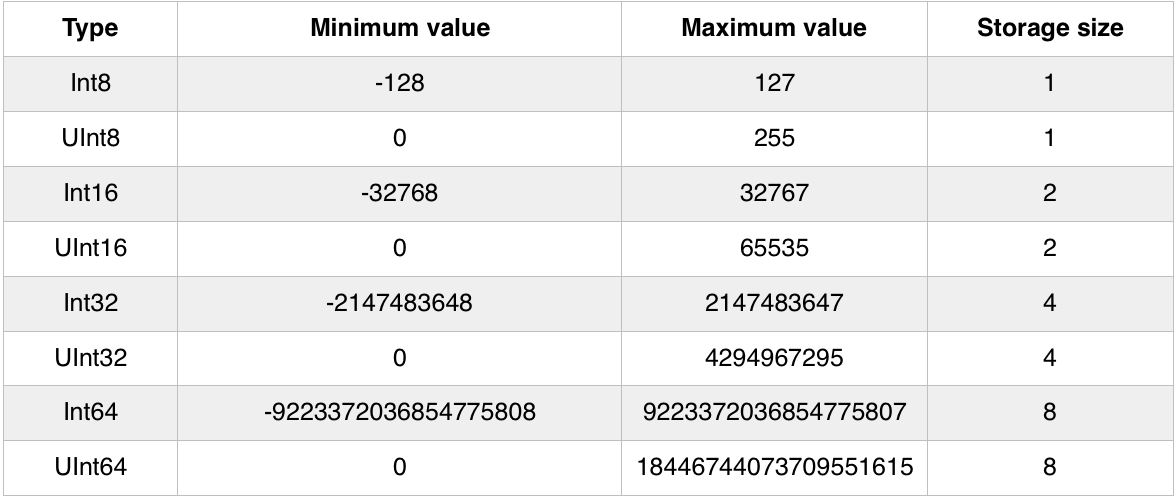You’ve been using `Double` to represent fractional numbers. Swift offers a `Float` type which has less range and precision than `Double` but requires half as much storage. Modern hardware has been optimized for `Double`, so it should be your go-to unless there is good reason to use a `Float`.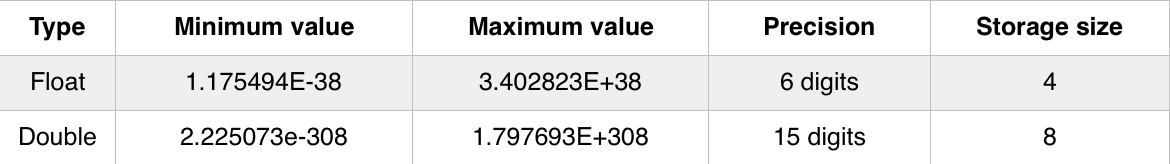Most of the time you will just use `Int` and `Double` to represent numbers, but you might encounter the other types every once in a while.

For example, suppose you need to add together an `Int16` with a `UInt8` and an `Int32`. You can do that like so:

``````let a: Int16 = 12
let b: UInt8 = 255
let c: Int32 = -100000

let answer = Int(a) + Int(b) + Int(c)  // answer is an Int
``````

## Type aliases

A useful feature of Swift is being able to create your own type which is actually an alias of another type. What this means you can do is give a more useful name to your type that describes what it is, but actually underneath it’s just another type. This is known as a type alias.

It’s simple to create a type alias, like so:

``````typealias Animal = String
``````

This creates a new type called `Animal`. When the compiler sees this type it simply treats it as a `String`. Therefore you could do something like this:

``````let myPet: Animal = "Dog"
``````

This might not seem too useful right now, but sometimes types can become complex and creating an alias for them can give them a simpler and more explicit name. For example, you might do the following:

``````typealias Coordinates = (Int, Int)
let xy: Coordinates = (2, 4)
``````

This creates a type called `Coordinates` which is a tuple containing two `Int`s and then uses it.

As you see more and more Swift you’ll see how type aliases can be very powerful and simplify code.

## A peek behind the curtains: Protocols

Even though there are a dozen different numeric types, they are pretty easy to understand and use, because they all roughly support the same operations. In other words, once you know how to use an `Int`, using any one of the flavors is straight-forward.

One of Swift’s truly great features is that it formalizes the idea of type commonality using what are known as protocols. By learning the protocol, you instantly understand how an entire family of types using that protocol work.

In the case of integers, the functionality can be diagrammed like so: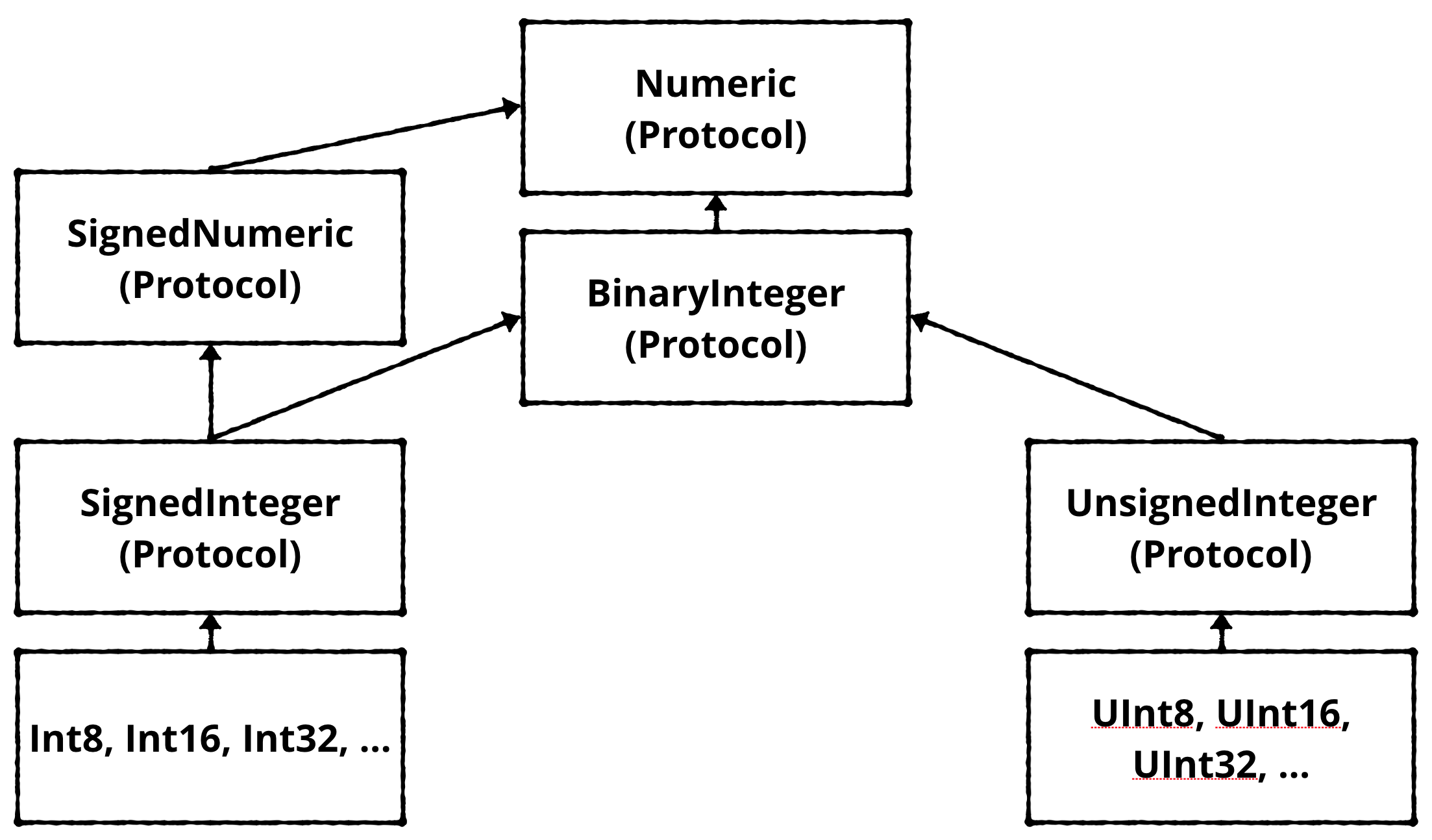The arrows indicate conformance to (sometimes called adoption of) a protocol. While this graph does not show all of the protocols that integer types conform to — it gives you insight about how things are organized.

Swift is the first protocol-based language. As you begin to understand the protocols that underly the types, you can leverage the system in ways not possible with other languages.

By the end of this book, you’ll be hooking into existing protocols and even creating new ones of your own.

## Challenges

Before moving on, here are some challenges to test your knowledge of types and operations. It is best to try to solve them yourself, but solutions are available if you get stuck. These came with the download or are available at the printed book’s source code link listed in the introduction.

### Challenge 1: Coordinates

Create a constant called `coordinates` and assign a tuple containing two and three to it.

### Challenge 2: Named coordinate

Create a constant called `namedCoordinate` with a `row` and `column` component.

### Challenge 3: Which are valid?

Which of the following are valid statements?

``````let character: Character = "Dog"
let character: Character = "🐶"
let string: String = "Dog"
let string: String = "🐶"
``````

### Challenge 4. Does it compile?

``````let tuple = (day: 15, month: 8, year: 2015)
let day = tuple.Day
``````

### Challenge 5: Find the error

What is wrong with the following code?

``````let name = "Matt"
name += " Galloway"
``````

### Challenge 6: What is the type of `value`?

What is the type of the constant named `value`?

``````let tuple = (100, 1.5, 10)
let value = tuple.1
``````

### Challenge 7: What is the value of month?

What is the value of the constant named `month`?

``````let tuple = (day: 15, month: 8, year: 2015)
let month = tuple.month
``````

### Challenge 8: What is the value of `summary`?

What is the value of the constant named `summary`?

``````let number = 10
let multiplier = 5
let summary = "\(number) multiplied by \(multiplier) equals \(number * multiplier)"
``````

### Challenge 9: Compute the value

What is the sum of `a` and `b`, minus `c`?

``````let a = 4
let b: Int32 = 100
let c: UInt8 = 12
``````

### Challenge 10: Different precision 𝜋s

What is the numeric difference between `Double.pi` and `Float.pi`?

## Key points

• Type conversion allows you to convert values of one type into another.
• Type conversion is required when using an operator, such as the basic arithmetic operators (`+`, `-`, `*`, `/`), with mixed types.
• Type inference allows you to omit the type when Swift already knows it.
• Unicode is the standard for mapping characters to numbers.
• A single mapping in Unicode is called a code point.
• The `Character` data type stores single characters. The `String` data type stores collections of characters, or strings.
• You can combine strings by using the addition operator.
• You can use string interpolation to build a string in-place.
• You can use tuples to group data into a single data type.
• Tuples can either be unnamed or named. Their elements are accessed with index numbers for unnamed tuples, or programmer given names for named tuples.
• There are many kinds of numeric types with different storage and precision capabilities.
• Type aliases can be used to create a new type that is simply a new name for another type.
• Protocols are how types are organized in Swift. They describe the common operations that multiple types share.

Have a technical question? Want to report a bug? You can ask questions and report bugs to the book authors in our official book forum here.

Have feedback to share about the online reading experience? If you have feedback about the UI, UX, highlighting, or other features of our online readers, you can send them to the design team with the form below:

© 2021 Razeware LLC